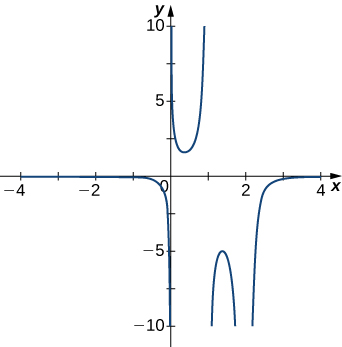$$\newcommand{\id}{\mathrm{id}}$$ $$\newcommand{\Span}{\mathrm{span}}$$ $$\newcommand{\kernel}{\mathrm{null}\,}$$ $$\newcommand{\range}{\mathrm{range}\,}$$ $$\newcommand{\RealPart}{\mathrm{Re}}$$ $$\newcommand{\ImaginaryPart}{\mathrm{Im}}$$ $$\newcommand{\Argument}{\mathrm{Arg}}$$ $$\newcommand{\norm}{\| #1 \|}$$ $$\newcommand{\inner}{\langle #1, #2 \rangle}$$ $$\newcommand{\Span}{\mathrm{span}}$$

# 4.6E: Exercises for Section 4.6

$$\newcommand{\vecs}{\overset { \rightharpoonup} {\mathbf{#1}} }$$ $$\newcommand{\vecd}{\overset{-\!-\!\rightharpoonup}{\vphantom{a}\smash {#1}}}$$$$\newcommand{\id}{\mathrm{id}}$$ $$\newcommand{\Span}{\mathrm{span}}$$ $$\newcommand{\kernel}{\mathrm{null}\,}$$ $$\newcommand{\range}{\mathrm{range}\,}$$ $$\newcommand{\RealPart}{\mathrm{Re}}$$ $$\newcommand{\ImaginaryPart}{\mathrm{Im}}$$ $$\newcommand{\Argument}{\mathrm{Arg}}$$ $$\newcommand{\norm}{\| #1 \|}$$ $$\newcommand{\inner}{\langle #1, #2 \rangle}$$ $$\newcommand{\Span}{\mathrm{span}}$$ $$\newcommand{\id}{\mathrm{id}}$$ $$\newcommand{\Span}{\mathrm{span}}$$ $$\newcommand{\kernel}{\mathrm{null}\,}$$ $$\newcommand{\range}{\mathrm{range}\,}$$ $$\newcommand{\RealPart}{\mathrm{Re}}$$ $$\newcommand{\ImaginaryPart}{\mathrm{Im}}$$ $$\newcommand{\Argument}{\mathrm{Arg}}$$ $$\newcommand{\norm}{\| #1 \|}$$ $$\newcommand{\inner}{\langle #1, #2 \rangle}$$ $$\newcommand{\Span}{\mathrm{span}}$$

For exercises 1 - 5, examine the graphs. Identify where the vertical asymptotes are located.

1)$$x=1$$

2)3)$$x=−1,\;x=2$$

4)5)$$x=0$$

For the functions $$f(x)$$ in exercises 6 - 10, determine whether there is an asymptote at $$x=a$$. Justify your answer without graphing on a calculator.

6) $$f(x)=\dfrac{x+1}{x^2+5x+4},\quad a=−1$$

7) $$f(x)=\dfrac{x}{x−2},\quad a=2$$

Yes, there is a vertical asymptote at $$x = 2$$.

8) $$f(x)=(x+2)^{3/2},\quad a=−2$$

9) $$f(x)=(x−1)^{−1/3},\quad a=1$$

Yes, there is vertical asymptote at $$x = 1$$.

10) $$f(x)=1+x^{−2/5},\quad a=1$$

In exercises 11 - 20, evaluate the limit.

11) $$\displaystyle \lim_{x→∞}\frac{1}{3x+6}$$

$$\displaystyle \lim_{x→∞}\frac{1}{3x+6} = 0$$

12) $$\displaystyle \lim_{x→∞}\frac{2x−5}{4x}$$

13) $$\displaystyle \lim_{x→∞}\frac{x^2−2x+5}{x+2}$$

$$\displaystyle \lim_{x→∞}\frac{x^2−2x+5}{x+2} = ∞$$

14) $$\displaystyle \lim_{x→−∞}\frac{3x^3−2x}{x^2+2x+8}$$

15) $$\displaystyle \lim_{x→−∞}\frac{x^4−4x^3+1}{2−2x^2−7x^4}$$

$$\displaystyle \lim_{x→−∞}\frac{x^4−4x^3+1}{2−2x^2−7x^4} = −\frac{1}{7}$$

16) $$\displaystyle \lim_{x→∞}\frac{3x}{\sqrt{x^2+1}}$$

17) $$\displaystyle \lim_{x→−∞}\frac{\sqrt{4x^2−1}}{x+2}$$

$$\displaystyle \lim_{x→−∞}\frac{\sqrt{4x^2−1}}{x+2} = -2$$

18) $$\displaystyle \lim_{x→∞}\frac{4x}{\sqrt{x^2−1}}$$

19) $$\displaystyle \lim_{x→−∞}\frac{4x}{\sqrt{x^2−1}}$$

$$\displaystyle \lim_{x→−∞}\frac{4x}{\sqrt{x^2−1}} = -4$$

20) $$\displaystyle \lim_{x→∞}\frac{2\sqrt{x}}{x−\sqrt{x}+1}$$

For exercises 21 - 25, find the horizontal and vertical asymptotes.

21) $$f(x)=x−\dfrac{9}{x}$$

Horizontal: none,
Vertical: $$x=0$$

22) $$f(x)=\dfrac{1}{1−x^2}$$

23) $$f(x)=\dfrac{x^3}{4−x^2}$$

Horizontal: none,
Vertical: $$x=±2$$

24) $$f(x)=\dfrac{x^2+3}{x^2+1}$$

25) $$f(x)=\sin(x)\sin(2x)$$

Horizontal: none,
Vertical: none

26) $$f(x)=\cos x+\cos(3x)+\cos(5x)$$

27) $$f(x)=\dfrac{x\sin(x)}{x^2−1}$$

Horizontal: $$y=0,$$
Vertical: $$x=±1$$

28) $$f(x)=\dfrac{x}{\sin(x)}$$

29) $$f(x)=\dfrac{1}{x^3+x^2}$$

Horizontal: $$y=0,$$
Vertical: $$x=0$$ and $$x=−1$$

30) $$f(x)=\dfrac{1}{x−1}−2x$$

31) $$f(x)=\dfrac{x^3+1}{x^3−1}$$

Horizontal: $$y=1,$$
Vertical: $$x=1$$

32) $$f(x)=\dfrac{\sin x+\cos x}{\sin x−\cos x}$$

33) $$f(x)=x−\sin x$$

Horizontal: none,
Vertical: none

34) $$f(x)=\dfrac{1}{x}−\sqrt{x}$$

For exercises 35 - 38, construct a function $$f(x)$$ that has the given asymptotes.

35) $$x=1$$ and $$y=2$$

Answers will vary, for example: $$y=\dfrac{2x}{x−1}$$

36) $$x=1$$ and $$y=0$$

37) $$y=4, \;x=−1$$

Answers will vary, for example: $$y=\dfrac{4x}{x+1}$$

38) $$x=0$$

In exercises 39 - 43, graph the function on a graphing calculator on the window $$x=[−5,5]$$ and estimate the horizontal asymptote or limit. Then, calculate the actual horizontal asymptote or limit.

39) [T] $$f(x)=\dfrac{1}{x+10}$$

$$\displaystyle \lim_{x→∞}\frac{1}{x+10}=0$$ so $$f$$ has a horizontal asymptote of $$y=0$$.

40) [T] $$f(x)=\dfrac{x+1}{x^2+7x+6}$$

41) [T] $$\displaystyle \lim_{x→−∞}x^2+10x+25$$

$$\displaystyle \lim_{x→−∞}x^2+10x+25 = ∞$$

42) [T] $$\displaystyle \lim_{x→−∞}\frac{x+2}{x^2+7x+6}$$

43) [T] $$\displaystyle \lim_{x→∞}\frac{3x+2}{x+5}$$

$$\displaystyle \lim_{x→∞}\frac{3x+2}{x+5}=3$$ so this function has a horizontal asymptote of $$y=3$$.

In exercises 44 - 55, draw a graph of the functions without using a calculator. Be sure to notice all important features of the graph: local maxima and minima, inflection points, and asymptotic behavior.

44) $$y=3x^2+2x+4$$

45) $$y=x^3−3x^2+4$$46) $$y=\dfrac{2x+1}{x^2+6x+5}$$

47) $$y=\dfrac{x^3+4x^2+3x}{3x+9}$$48) $$y=\dfrac{x^2+x−2}{x^2−3x−4}$$

49) $$y=\sqrt{x^2−5x+4}$$50) $$y=2x\sqrt{16−x^2}$$

51) $$y=\dfrac{\cos x}{x}$$, on $$x=[−2π,2π]$$52) $$y=e^x−x^3$$

53) $$y=x\tan x, \quad x=[−π,π]$$54) $$y=x\ln(x), \quad x>0$$

55) $$y=x^2\sin(x),\quad x=[−2π,2π]$$56) For $$f(x)=\dfrac{P(x)}{Q(x)}$$ to have an asymptote at $$y=2$$ then the polynomials $$P(x)$$ and $$Q(x)$$ must have what relation?

57) For $$f(x)=\dfrac{P(x)}{Q(x)}$$ to have an asymptote at $$x=0$$, then the polynomials $$P(x)$$ and $$Q(x).$$ must have what relation?

$$Q(x).$$ must have have $$x^{k+1}$$ as a factor, where $$P(x)$$ has $$x^k$$ as a factor.

58) If $$f′(x)$$ has asymptotes at $$y=3$$ and $$x=1$$, then $$f(x)$$ has what asymptotes?

59) Both $$f(x)=\dfrac{1}{x−1}$$ and $$g(x)=\dfrac{1}{(x−1)^2}$$ have asymptotes at $$x=1$$ and $$y=0.$$ What is the most obvious difference between these two functions?

$$\displaystyle \lim_{x→1^−}f(x)=-\infty \text{ and } \lim_{x→1^−}g(x)=\infty$$